# How to remove a specific element from Queue

• Last Updated : 29 Sep, 2021

Given a queue q[] and an integer K, the task is to define a method to remove a specific element from the queue q[]. If there are multiple occurrences of element K, then, remove the first one from the queue q[].

Examples:

Input: q[] = {10, 20, 30, 40, 50, 60}, K = 30
Output: {10, 20, 40, 50, 60}
Explanation: After removal of 30, the queue becomes {10, 20, 40, 50, 60}.

Input: q[] = {1, 2, 3, 3}, K = 3
Output: {1, 2, 3}
Explanation: After removal of the first occurrence of 3, the queue becomes {1, 2,  3}.

Approach: The idea is to create a temporary queue ref[] and store all the elements in it, until K is found. Then, remove K from the original queue q[], and insert the remaining elements back into the queue q[]. Follow the steps below to solve the problem: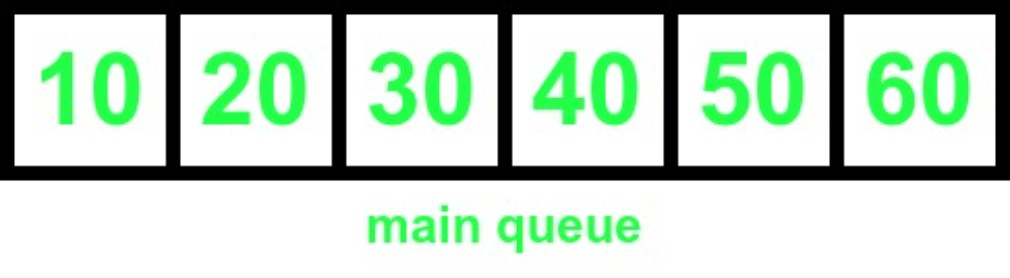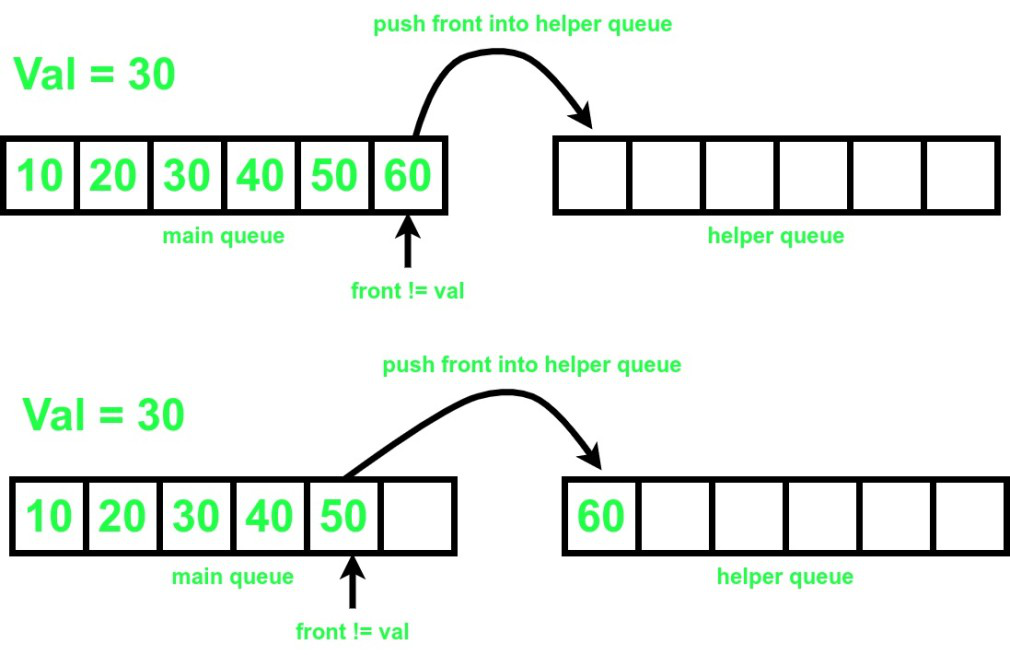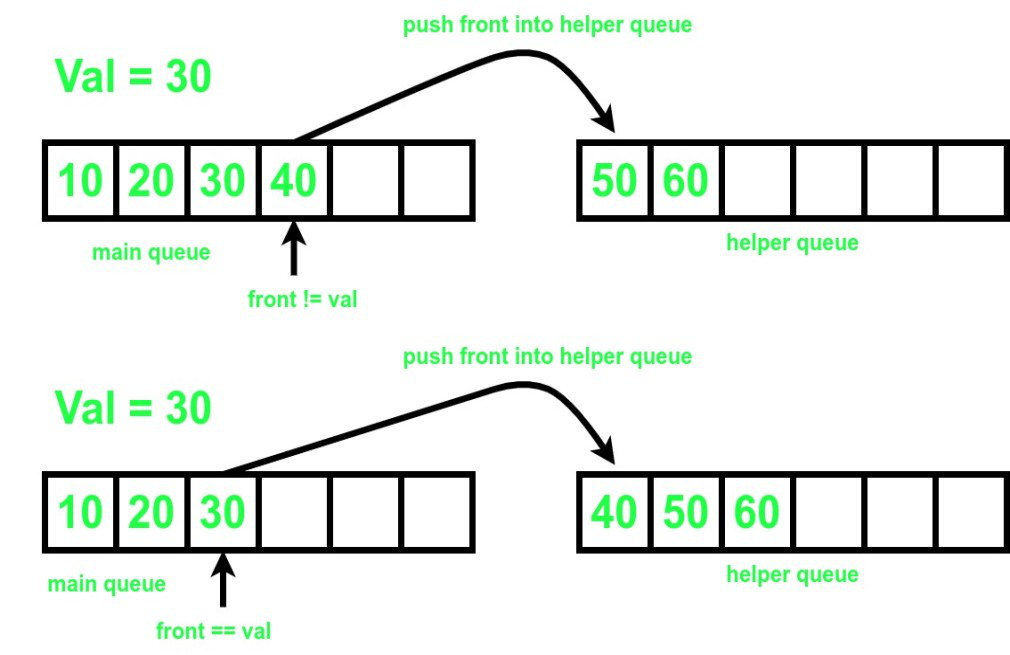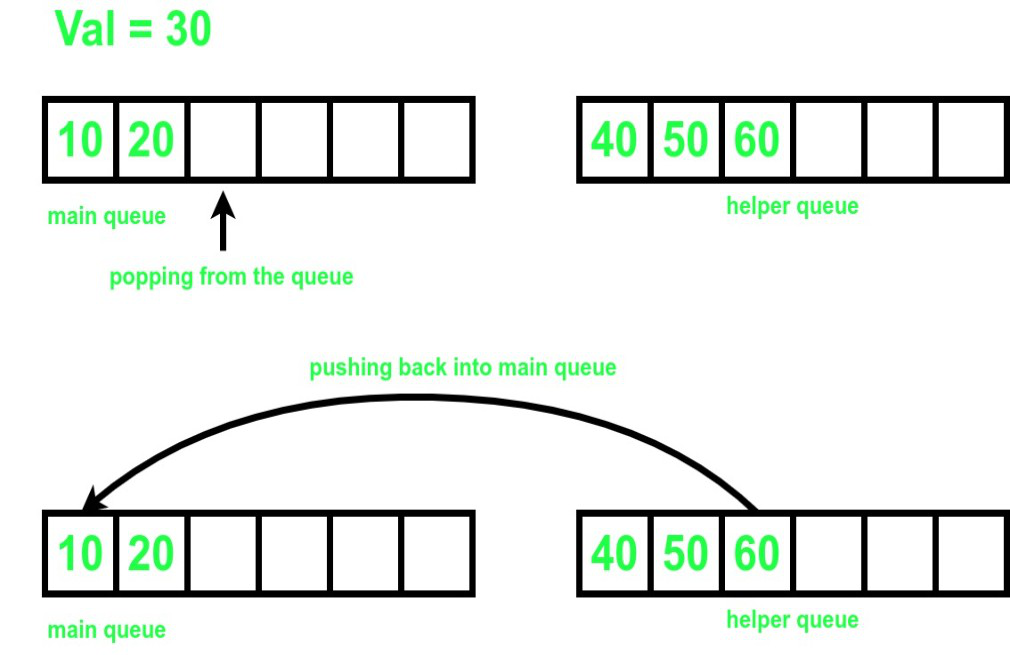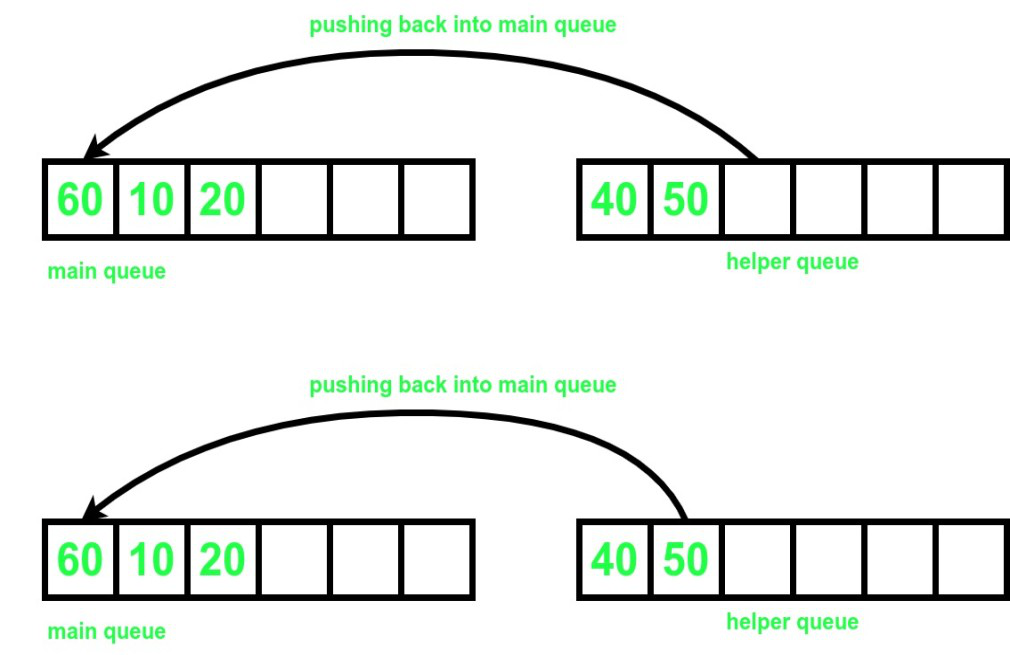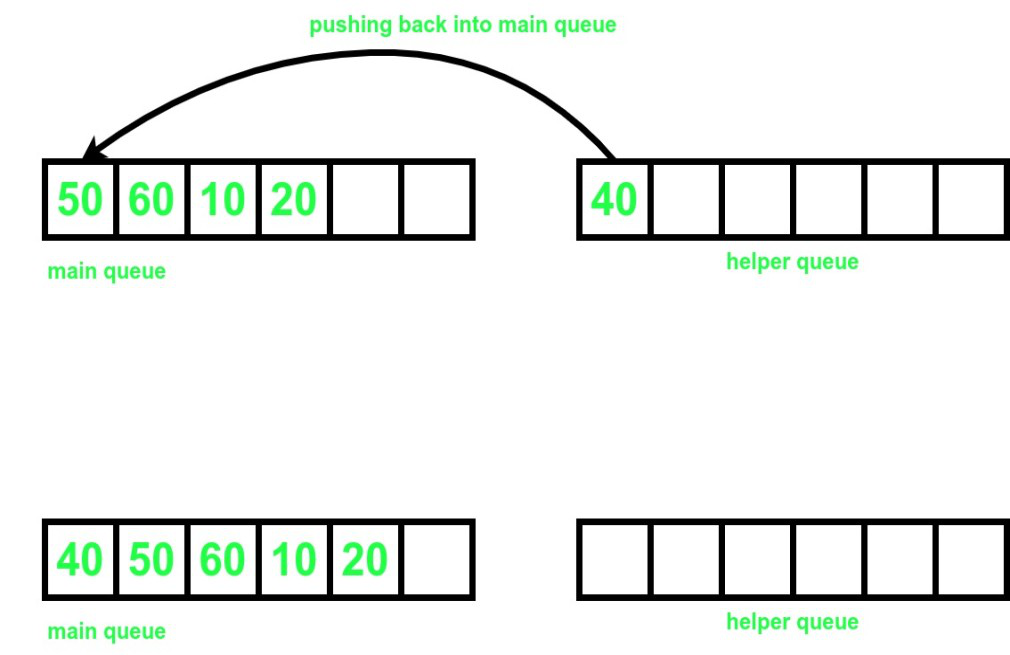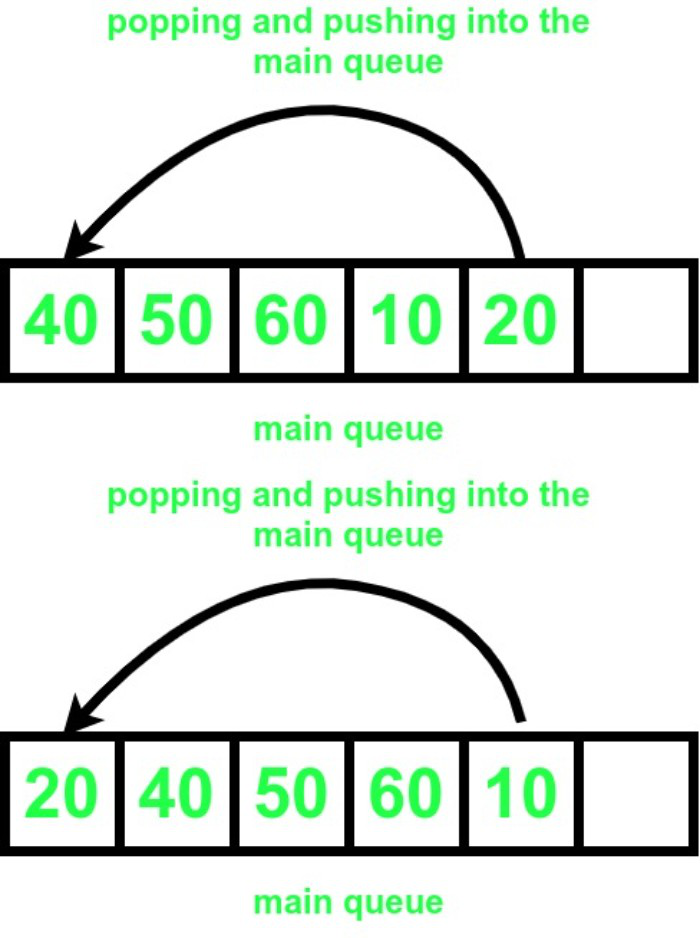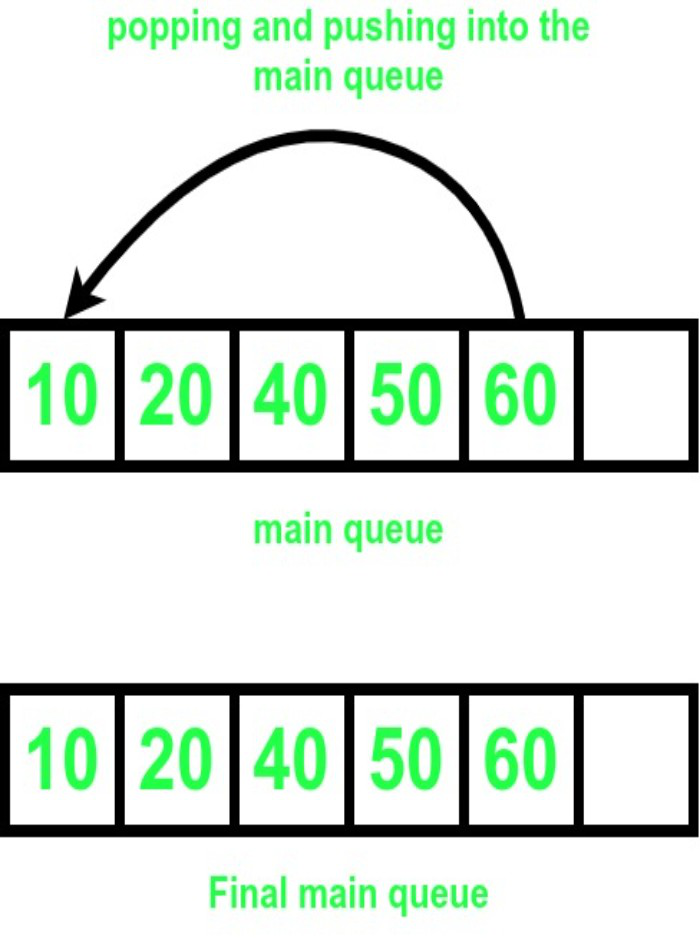Below is the implementation of the above approach.

## C++

 `// C++ program for the above approach.``#include ``using` `namespace` `std;` `// Function to remove an element from``// the queue``void` `remove``(``int` `t, queue<``int``>& q)``{` `    ``// Helper queue to store the elements``    ``// temporarily.``    ``queue<``int``> ref;``    ``int` `s = q.size();``    ``int` `cnt = 0;` `    ``// Finding the value to be removed``    ``while` `(q.front() != t and !q.empty()) {``        ``ref.push(q.front());``        ``q.pop();``        ``cnt++;``    ``}` `    ``// If element is not found``    ``if` `(q.empty()) {``        ``cout << ``"element not found!!"` `<< endl;``        ``while` `(!ref.empty()) {` `            ``// Pushing all the elements back into q``            ``q.push(ref.front());``            ``ref.pop();``        ``}``    ``}` `    ``// If element is found``    ``else` `{``        ``q.pop();``        ``while` `(!ref.empty()) {` `            ``// Pushing all the elements back into q``            ``q.push(ref.front());``            ``ref.pop();``        ``}``        ``int` `k = s - cnt - 1;``        ``while` `(k--) {` `            ``// Pushing elements from front of q to its back``            ``int` `p = q.front();``            ``q.pop();``            ``q.push(p);``        ``}``    ``}``}` `// Function to print all the elements``// of the queue.``void` `print(queue<``int``> qr)``{``    ``while` `(!qr.empty()) {``        ``cout << qr.front() << ``" "``;``        ``qr.pop();``    ``}``    ``cout << endl;``}` `// Driver Code``int` `main()``{``    ``queue<``int``> q;` `    ``// Pushing into the queue``    ``q.push(10);``    ``q.push(20);``    ``q.push(30);``    ``q.push(40);``    ``q.push(50);``    ``q.push(60);``    ``print(q);` `    ``// Removing 39 from the queue``    ``remove``(39, q);``    ``print(q);` `    ``// Removing 30 from the queue``    ``remove``(30, q);``    ``print(q);``    ``return` `0;``}`

## Java

 `// Java program for the above approach.` `import` `java.util.*;` `class` `GFG{` `// Function to remove an element from``// the queue``static` `Queue q;``static` `void` `remove(``int` `t)``{` `    ``// Helper queue to store the elements``    ``// temporarily.``    ``Queue ref = ``new` `LinkedList<>();``    ``int` `s = q.size();``    ``int` `cnt = ``0``;` `    ``// Finding the value to be removed``    ``while` `(!q.isEmpty() && q.peek() != t) {``        ``ref.add(q.peek());``        ``q.remove();``        ``cnt++;``    ``}` `    ``// If element is not found``    ``if` `(q.isEmpty()) {``        ``System.out.print(``"element not found!!"` `+``"\n"``);``        ``while` `(!ref.isEmpty()) {` `            ``// Pushing all the elements back into q``            ``q.add(ref.peek());``            ``ref.remove();``        ``}``    ``}` `    ``// If element is found``    ``else` `{``        ``q.remove();``        ``while` `(!ref.isEmpty()) {` `            ``// Pushing all the elements back into q``            ``q.add(ref.peek());``            ``ref.remove();``        ``}``        ``int` `k = s - cnt - ``1``;``        ``while` `(k-- >``0``) {` `            ``// Pushing elements from front of q to its back``            ``int` `p = q.peek();``            ``q.remove();``            ``q.add(p);``        ``}``    ``}``}` `// Function to print all the elements``// of the queue.``static` `void` `print()``{``    ``Queue qr = ``new` `LinkedList<>(q);``    ``while` `(!qr.isEmpty()) {``        ``System.out.print(qr.peek()+ ``" "``);``        ``qr.remove();``    ``}` `    ``System.out.println();``}` `// Driver Code``public` `static` `void` `main(String[] args)``{``    ``q = ``new` `LinkedList<>();` `    ``// Pushing into the queue``    ``q.add(``10``);``    ``q.add(``20``);``    ``q.add(``30``);``    ``q.add(``40``);``    ``q.add(``50``);``    ``q.add(``60``);``    ``print();` `    ``// Removing 39 from the queue``    ``remove(``39``);``    ``print();` `    ``// Removing 30 from the queue``    ``remove(``30``);``    ``print();``}``}` `// This code is contributed by 29AjayKumar`

## C#

 `// C# program for the above approach.``using` `System;``using` `System.Collections;``public` `class` `GFG{``    ` `      ``// Function to remove an element from``// the queue``static` `Queue q = ``new` `Queue();``static` `void` `remove_(``int` `t)``{` `    ``// Helper queue to store the elements``    ``// temporarily.``    ``Queue reff = ``new` `Queue();``    ``int` `s = q.Count;``    ``int` `cnt = 0;` `    ``// Finding the value to be removed``    ``while` `((``int``)q.Count != 0 && (``int``)q.Peek() != t) {``      ` `        ``reff.Enqueue(q.Peek());``        ``q.Dequeue();``        ``cnt++;``    ``}` `    ``// If element is not found``    ``if` `(q.Count == 0) {``        ``Console.WriteLine(``"element not found!!"``);``          ` `        ``while` `(reff.Count != 0) {``            ` `            ``// Pushing all the elements back into q``            ``q.Enqueue(reff.Peek());``            ``reff.Dequeue();``        ``}``    ``}` `    ``// If element is found``    ``else` `{``        ``q.Dequeue();``        ``while` `(reff.Count != 0) {` `            ``// Pushing all the elements back into q``            ``q.Enqueue(reff.Peek());``            ``reff.Dequeue();``        ``}``        ``int` `k = s - cnt - 1;``        ``while` `(k-- >0) {` `            ``// Pushing elements from front of q to its back``            ``int` `p = (``int``)q.Peek();``            ``q.Dequeue();``            ``q.Enqueue(p);``        ``}``    ``}``}` `// Function to print all the elements``// of the queue.``static` `void` `print()``{``    ``Queue qr = (Queue)q.Clone();``    ``while` `(qr.Count != 0) {``        ``Console.Write(qr.Peek()+ ``" "``);``        ``qr.Dequeue();``    ``}` `    ``Console.WriteLine();``}` `// Driver Code``static` `public` `void` `Main (){` `    ``// Pushing into the queue``    ``q.Enqueue(10);``    ``q.Enqueue(20);``    ``q.Enqueue(30);``    ``q.Enqueue(40);``    ``q.Enqueue(50);``    ``q.Enqueue(60);``  ` `    ``print();` `    ``// Removing 39 from the queue``    ``remove_(39);``    ``print();` `    ``// Removing 30 from the queue``    ``remove_(30);``    ``print();``}``}` `// This code is contributed by Dharanendra L V.`

Output

```10 20 30 40 50 60